## Integration

 3.1 Integration as the Inverse of Differentiation

Integration
 Integration is the reverse process of differentiation.

• A process is denoted by the symbol $$\int ... \,dx$$

Differentiation Integration
 $$\dfrac{d}{dx}[f(x)] = f'(x)$$
 $$\int f'(x) \ dx = f(x)$$

• In general,
 If $$\dfrac{d}{dx}[f(x)] = f'(x)$$, then the integral of $$f'(x)$$ with respct to $$x$$ is $$\int f'(x) \ dx = f(x)$$.

 Example Given $$\dfrac{d}{dx} (4x^2) = 8x$$, find $$\int 8x \ dx$$. Solution: Differentiation of $$4x^2$$ is $$8x$$. By the reverse of differentiation, the integration of $$8x$$ is $$4x^2$$. Hence, $$\int 8x \ dx = 4x^2$$.

 3.2 Indefinite Integral

• For a constant $$a$$,
 $$\int a \ dx = ax +c$$, where $$a$$ and $$c$$ are constants.

• For a function $$ax^n$$,
 $$\int ax^n \ dx = \dfrac{ax^{n+1}}{n+1}$$, where $$a$$ and $$c$$ are constants, $$n$$ is an integer and $$n \neq -1$$.

• The function $$ax+c$$  and  $$\dfrac{ax^{n+1}}{n+1} +c$$  are known as indefinite integrals for a constant $$a$$ with respect to $$x$$ and function $$ax^n$$ with respect to $$x$$ respectively

Constant of Integration, $$c$$
 The constant of integration, $$c$$ in an indefinite integrals are different and is added as part of indefinite integral for a function such as: $$\int 5 \ dx = 5x +c$$

Remark
 $$\int ax^n \ dx = a \int x^n \ dx$$

Example

Integrate each of the following with respect to $$x$$ :

 (a) $$-0.5$$ (b) $$\int \dfrac{2}{x^2} \ dx$$ Solution: (a) $$\int -0.5 \ dx = -0.5x +c$$ (b) \begin{aligned} \int \dfrac{2}{x^2} \ dx &= 2 \int x^{-2} \ dx\\\\ &= 2 \begin{pmatrix} \dfrac{x^{-2+1}}{-2+1} \end{pmatrix} +c\\\\ &= -2x^{-1} +c\\\\ &= -\dfrac{2}{x} +c \end{aligned}

• If $$f(x)$$ and $$g(x)$$ are functions, then
 $$\int [f(x) \pm g(x)] \ dx = \int f(x) \ dx \pm g(x) \ dx$$

 Example Find the integral for the following $$\int (x-2)(x+6) \ dx$$ Solution: \begin{aligned} \int (x-2)(x+6) \ dx &= \int (x^2 +4x - 12) \ dx\\\\ &= \int x^2 \ dx + \int 4x \ dx-\int12 \ dx\\\\ &= \dfrac{x^3}{3} + \dfrac{4x^2}{2} - 12x +c\\\\ &= \dfrac{x^3}{3} + 2x^2- 12x +c \end{aligned}

• Substitution method can be used for function $$(ax+b)^n$$, where $$a$$ and $$b$$ are constants, $$n$$ is an integer and $$n \neq -1$$
 $$\int (ax+b)^n \ dx = \dfrac{(ax+b)^{n+1}}{a(n+1)} +c$$, where $$a$$ and $$b$$ are constants, $$n$$ is an integer and $$n \neq -1$$.

• In general,
 Given the gradien function $$\dfrac{dy}{dx} = f'(x)$$, the equation of curve for the function is $$y = \int f'(x) \ dx$$.

Example

 (a) By using the substitution method, find the indefinite integral for the following $$\int \sqrt{5x+2} \ dx$$ (b) The gradient function of a curve at point $$(x, y)$$ is given by $$\dfrac{dy}{dx} = 15x^2 + 4x- 3$$. If the curve passes through the point $$(-1, 2)$$,  find the equation of the curve. Solution: (a) Let $$u=5x+2$$, then, \begin{aligned} \dfrac{dy}{dx} &=5\\\\ dx&= \dfrac{du}{5} \end{aligned} \begin{aligned} \int \sqrt{5x+2} \ dx &= \int \dfrac{\sqrt u}{5} \ du\\\\ &= \int \dfrac{u^{\frac{1}{2}}}{5} \ du\\\\ &=\dfrac{2}{15}u^{\frac{3}{2}} +c\\\\ &= \dfrac{2}{15}(5x+2)^{\frac{3}{2}}+c \end{aligned} (b) Given $$\dfrac{dy}{dx} = 15x^2 + 4x- 3$$, Then, \begin{aligned} y&=\int (15x^2 +4x-3) \ dx\\\\ y&=5x^3 +2x^2-3x+c \end{aligned} When $$x=-1$$ and $$y=2$$, \begin{aligned} 2&=5(-1)^3+2(-1)^2-3(-1)+c\\ c&=2 \end{aligned} Thus, the equation of the curve is $$y=5x^3 +2x^2-3x+2$$

 3.3 Definite Integral

• The definite integral of a function $$f(x)$$ with respect to $$x$$ with the interval from $$x=a \text{ to } x=b$$ can be written as:
 \begin{aligned} \int_{a}^{b} f(x) \ dx &= [g(x)+c]_{a}^{b} \\ &=[g(b)+c]-[g(a)+c]\\ &=g(b)-g(a) \end{aligned}

Characteristics of Definite Integrals

For the function $$f(x)$$ and $$g(x)$$,

 (a) $$\int_{a}^{b} f(x) \ dx = 0$$ (b) $$\int_{a}^{b} f(x) \ dx = -\int_{a}^{b} f(x) \ dx$$ (c) $$\int_{a}^{b} kf(x) \ dx = k\int_{a}^{b} f(x) \ dx$$, where $$k$$ is a constant (d) $$\int_{a}^{b} f(x) \ dx + \int_{b}^{c} f(x) \ dx = \int_{a}^{c} f(x) \ dx$$, where $$a (e) \(\int_{a}^{b} [f(x) \pm g(x)] \ dx = \int_{a}^{b} f(x) \ dx \pm \int_{a}^{b}g(x) \ dx$$

• Area of a region between the curve and the $$x$$-axis :
 $$A = \int_{a}^{b} y \ dx$$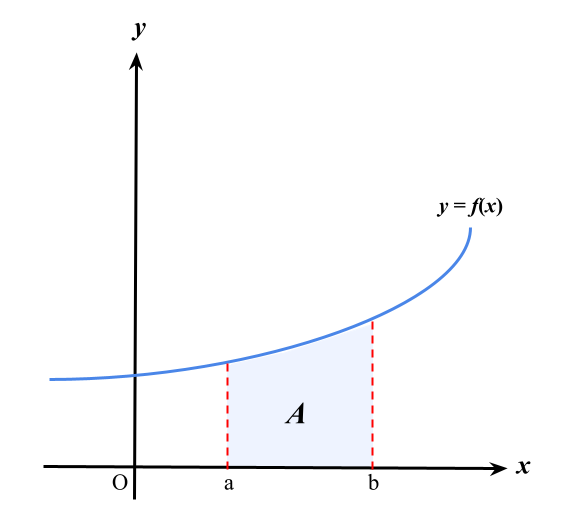• For the value of the area bounded by the curve and the $$x$$-axis,

 1. If the region is below the $$x$$-axis, then the integral value is negative 2. If the region is above the $$x$$-axis, the the integral value is positive 3. The areas of both regions are positive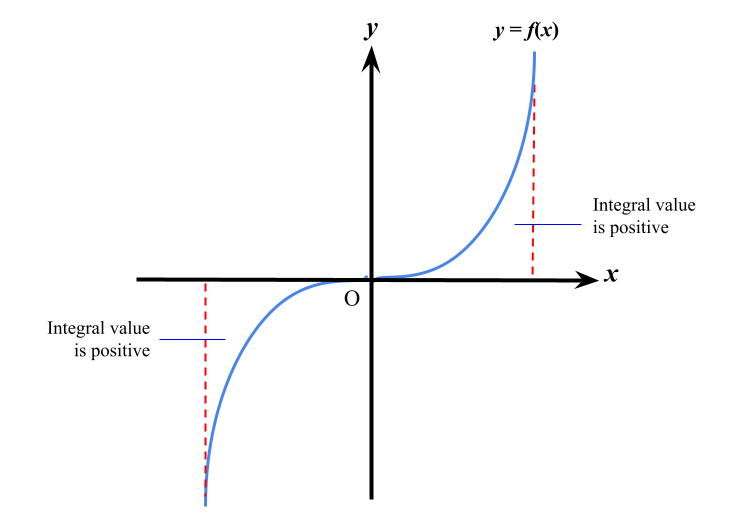• Area between the curve and the $$y$$-axis :
 $$A= \int_{a}^{b} x \ dy$$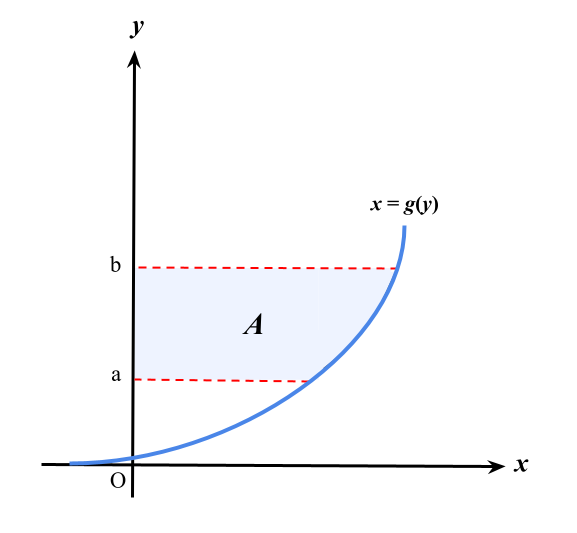• For a region bounded by the curve and the $$y$$-axis,

 1. If the region is to the left of $$y$$-axis, then the integral value is negative 2. If the region is to the right of $$y$$-axis, then the integral value is positive 3. The areas of both regions are positive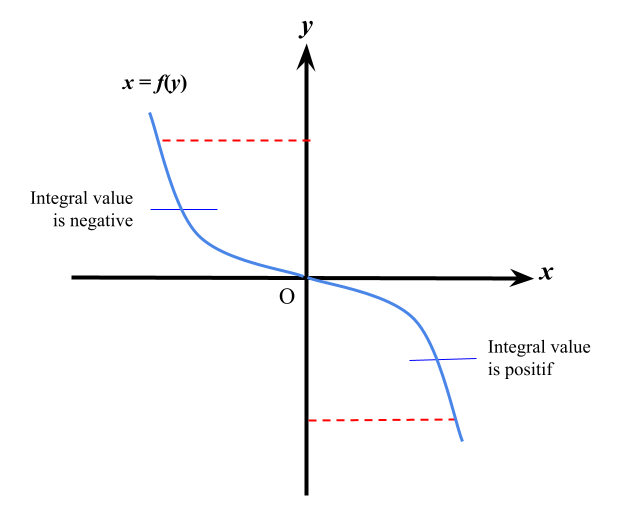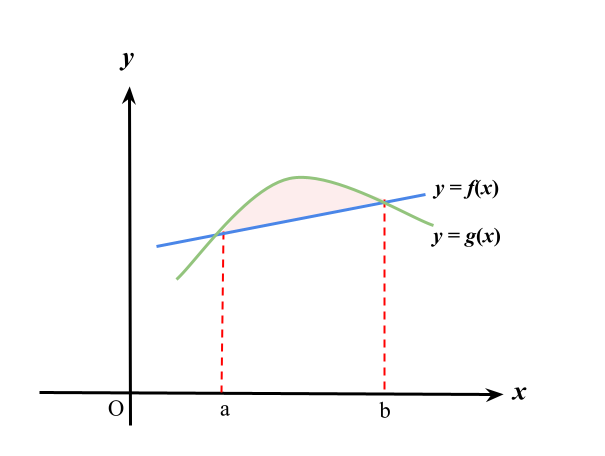• Area between the curve and a straight line:
 \begin{aligned} \text{The area of shaded region }&= \int_{a}^{b} g(x) \ dx -\int_{a}^{b} f(x) \ dx \\\\ &=\int_{a}^{b} [g(x)-f(x)] \ dx \end{aligned}

• To find the area, make sure that the function of the curve or line on the top is substracted to the function of the curve or line at the bottom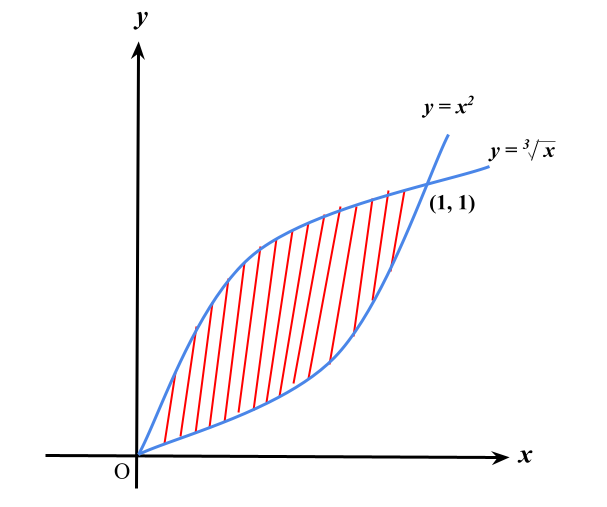Example The curves $$y=x^2$$ and $$y= \sqrt {x}$$ intersect at points $$(0,0) \text{ and } (1,1)$$. Find the area between the two curves. Solution: \begin{aligned} \text{Area of the region }&= \int_{0}^{1} \sqrt x \ dx - \int_{0}^{1} x^2 \ dx\\\\ &= \int_{0}^{1} (x^{\frac{1}{3}}-x^2) \ dx\\\\ &=\begin{bmatrix} \dfrac{3x^{\frac{4}{3}}}{4}-\dfrac{x^3}{3} \end{bmatrix}_{0}^{1}\\\\ &=\begin{bmatrix} \dfrac{3(1)^{\frac{4}{3}}}{4}-\dfrac{1^3}{3} \end{bmatrix}_{0}^{1} - \begin{bmatrix} \dfrac{3(0)^{\frac{4}{3}}}{4}-\dfrac{0^3}{3} \end{bmatrix}_{0}^{1}\\\\ &=\dfrac{5}{12} \text{ unit}^2 \end{aligned}

• The generated volume of a solid when an area is rotated through $$360^\text{o}$$ about the $$x$$-axis or the $$y$$-axis
• The value of generated volume is always positive

• Volume when a region is rotated through $$360^\text{o}$$ about the $$x$$-axis :
 $$V= \int_{a}^{b} \pi y^2 \ dx$$

• Volume when a region is rotated through $$360^\text{o}$$ about the $$y$$-axis :
 $$V = \int_{a}^{b} \pi x^2 \ dy$$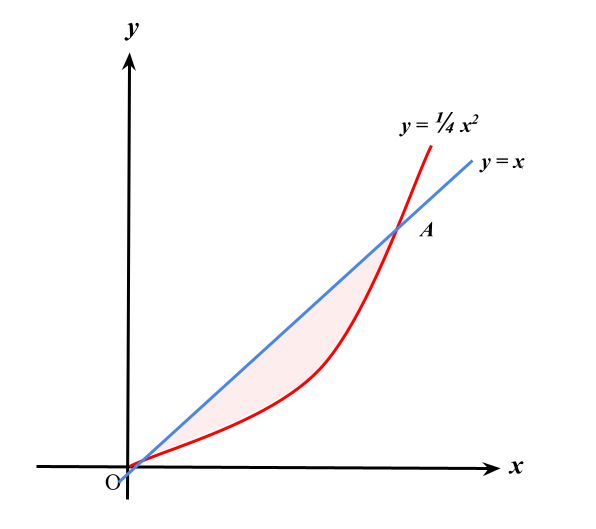Example

Based on the diagram above, the curve $$y=\dfrac{1}{4}x^2$$ intersects the stright line  $$y=x \text{ at } O(0,0) \text{ and }A(4,4)$$.

Find the generated volume, in terms of $$\pi$$, when the shaded region is revolved fully about the $$x$$-axis.

Solution:

Let $$V_{1}$$ the volume generated by the straight line $$y=x$$ and $$V_{2}$$ be the volume generated by the curve  $$y=\dfrac{1}{4}x^2$$ from $$x=0 \text{ to }x=4$$.

 \begin{aligned} V_1 &= \int_{0}^{4} \pi (x)^2 \ dx\\\\ &=\pi \int_{0}^{4} (x)^2 \ dx\\\\ &= \pi \begin{bmatrix} \dfrac{x^3}{3} \end{bmatrix}_{0}^{4}\\\\ &= \pi \begin{bmatrix} \dfrac{4^3}{3} - \dfrac{0^3}{3} \end{bmatrix} \\\\ &=\dfrac{64}{3}\pi \text{ unit}^3 \end{aligned} \begin{aligned} V_2 &= \int_{0}^{4} \pi \begin{pmatrix} \dfrac{1}{4}x^2 \end{pmatrix}^2 \ dx\\\\ &=\pi \int_{0}^{4} \dfrac{1}{16}x^4 \ dx\\\\ &= \pi \begin{bmatrix} \dfrac{x^5}{16(5)} \end{bmatrix}_{0}^{4}\\\\ &= \pi \begin{bmatrix} \dfrac{4^5}{80} - \dfrac{0^5}{80} \end{bmatrix} \\\\ &=\dfrac{64}{5}\pi \text{ unit}^3 \end{aligned}

Thus, the generated volume

\begin{aligned} &= V_1 - V_2\\\\ &=\dfrac{64}{3} \pi -\dfrac{64}{5} \pi\\\\ &=8\dfrac{8}{15}\pi \text{ unit}^3 \end{aligned}

 3.4 Application of Integration
 Integration can be used to find the areas of regions formed by curves Determine the distance moved by an object from its velocity function Solve other types of problem in various fields such as economics, biology and statistics